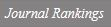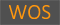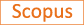Infinite families of irregular primes in cyclotomic function fields

Title
Infinite families of irregular primes in cyclotomic function fields
Authors
Lee, JungyunLee, Yoonjin
Ewha Authors
SCOPUS Author ID
이윤진Issue Date
2020
Journal Title
JOURNAL OF NUMBER THEORY
ISSN
0022-314X1096-1658Citation
JOURNAL OF NUMBER THEORY vol. 207, pp. 1 - 21
Keywords
Irregular primeRegulatorFunction fieldSextic extension
Publisher
Indexed
SCI; SCIE; SCOPUSDocument Type
Article
Abstract
We find both a lower bound and an upper bound on the p-rank of the divisor class group of the fth cyclotomic function field k(Lambda(f)) and the Jacobian of k(Lambda(f))(F) over bar (q), where f is an irreducible polynomial in the rational function field k = F-q(t) and F-q is the finite field of order q with characteristic p. Moreover, we find two types of infinite families of irregular primes f for which the divisor class numbers of the maximal real cyclotomic function fields k(Lambda(f))(+) with conductor f are divisible by N. For the first family of irregular primes, N is equal to p(p(p-1)), a power of a prime, and for the second family of irregular primes, N is a composite number (pl)(5) for a prime l different from a prime p. Furthermore, in the former case, the divisor class group of k(Lambda(f))(+) has p-rank at least p(p-1). (C) 2018 Published by Elsevier Inc.
DOI
10.1016/j.jnt.2018.09.008
Appears in Collections:
자연과학대학 > 수학전공 > Journal papers
Files in This Item:
There are no files associated with this item.
Export
RIS (EndNote)
XLS (Excel)
XML